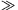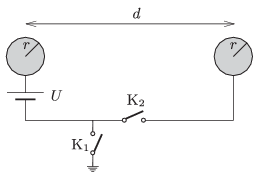Mathematical and Physical Journal
for High Schools
Issued by the MATFUND Foundation
 Already signed up? New to KöMaL?

#Problem P. 4626. (March 2014)

P. 4626. There are two alike initially uncharged spheres of radius r at a distance of dr, as shown in the figure.a) Switchis closed. What is the force exerted between the two spheres?

b) What would be the force between the two spheres, if in the previous experiment instead of closing switch, switchwas closed?

c) Determine the force exerted between the spheres if both switches are closed.

(6 pont)

Deadline expired on April 10, 2014.

Sorry, the solution is available only in Hungarian. Google translation

Megoldásvázlat. Mindhárom esetben vonzóerő alakul ki:

$\displaystyle F_{(a)}=\frac{U^2}{4k}\left(\frac{r}{d}\right)^2,\quad F_{(b)}=\frac{2U^2}{ k}\left(\frac{r}{d}\right)^5,\quad F_{(c)}=\frac{U^2}{k}\left(\frac{r}{d}\right)^3.$

### Statistics:

 21 students sent a solution. 6 points: Fehér Zsombor, Horicsányi Attila, Janzer Barnabás, Sal Kristóf. 5 points: Antalicz Balázs, Dinev Georgi. 4 points: 4 students. 3 points: 3 students. 2 points: 5 students. 1 point: 2 students. 0 point: 1 student.

Problems in Physics of KöMaL, March 2014